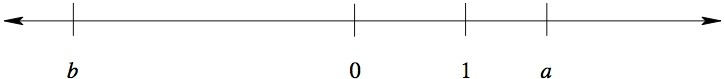# Operations on the number line

Alignments to Content Standards: 7.NS.A.1

A number line is shown below. The numbers $0$ and $1$ are marked on the line, as are two other numbers $a$ and $b$.Which of the following numbers is negative? Choose all that apply. Explain your reasoning.

1. $a - 1$
2. $a - 2$
3. $-b$
4. $a + b$
5. $a - b$
6. $ab + 1$

## IM Commentary

There is a distinction in the Common Core State Standards between a fraction and a rational number. Fractions are always positive, and when thinking of the symbol $\frac{a}{b}$ as a fraction, it is possible to interpret it as $a$ equal-sized pieces where $b$ pieces make one whole. The rational numbers are the set of fractions taken together with their opposites: understanding rational numbers requires understanding both fractions and signed numbers. The standard 7.NS.1 signals a significant shift from working exclusively with positive numbers to working with signed numbers. The focus of this task is on the nature of signed numbers rather than the "part-whole" interpretation of fractions.

The purpose of this task is to help solidify students' understanding of signed numbers as points on a number line and to understand the geometric interpretation of adding and subtracting signed numbers. This task (like all tasks featured on the Illustrative Mathematics website) assumes that the number line is drawn to scale.

## Solution

1. $a$ is greater than $1$, so $a - 1$ is positive.

2. The distance between $a$ and $1$ appears to be less than the distance between $1$ and $0$, so it looks like $a$ is less than $2$. Thus $a-2$ is negative.

3. $b$ is negative, so $-b$ is positive.

4. The distance between $a$ and $0$ appears to be less than the distance between $b$ and $0$, so it looks like $|a|$ is less than $|b|$. Since $b$ is negative and a is positive, $a+b$ is negative.

5. $a-b$ = $a+ -b$. Since $b$ is negative, $-b$ is positive. $a$ is also positive. Thus, $a-b$ is positive.

6. Since $|a|$ and $|b|$ are both greater than $1$, $|ab|$ is also greater than 1 (this builds on the intuition students gained in fifth grade as in 5.NF.5). $ab$ is negative since $a$ is positive and $b$ is negative. Thus, $ab+1$ is negative.